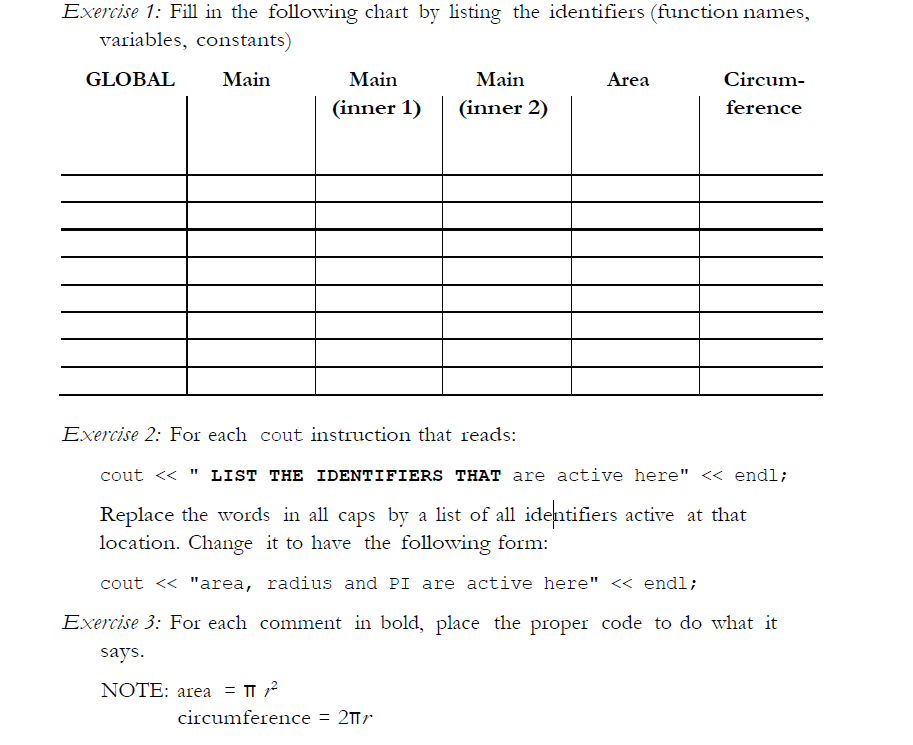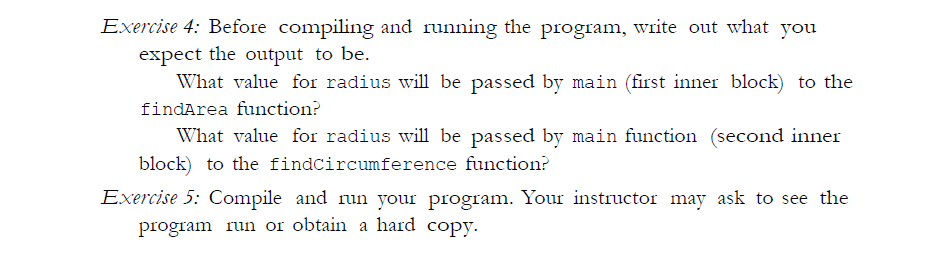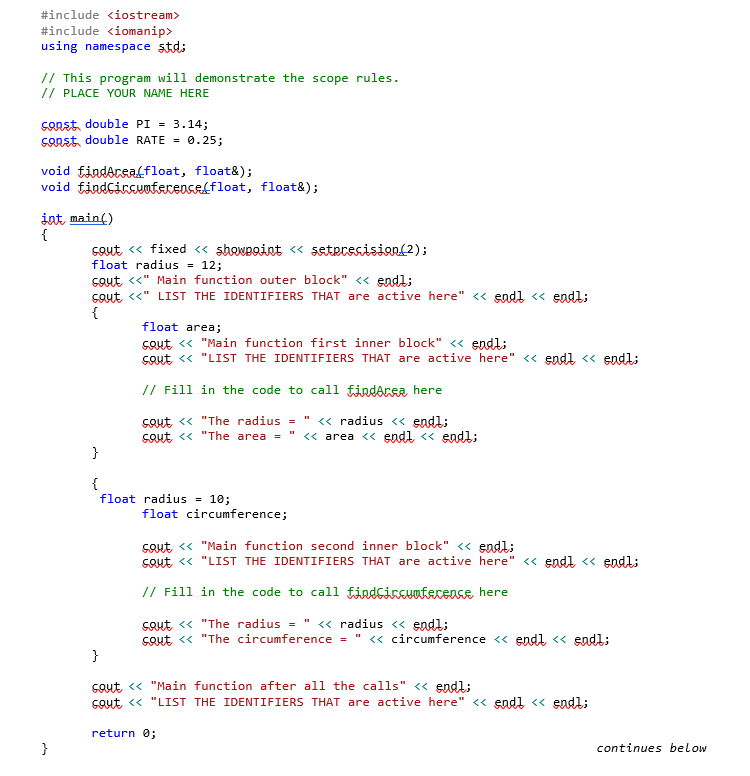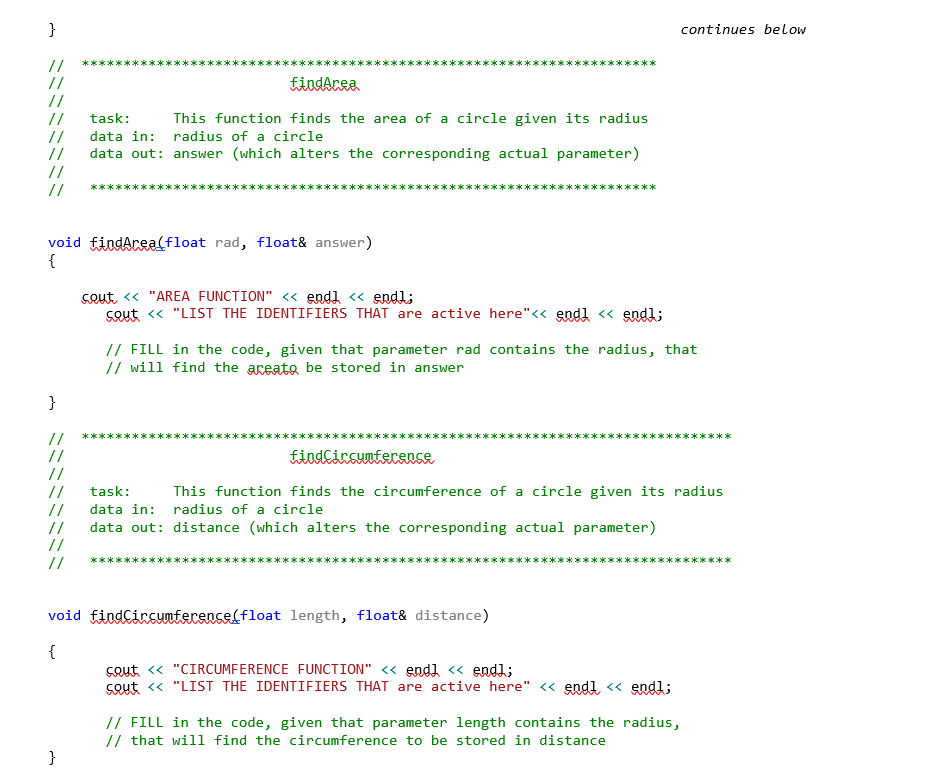# Answered Essay: I need to complete these exercises with a given code. Every instruction is included in the pictures

I need to complete these exercises with a given code. Every instruction is included in the pictures.The code I need to modify with the exercises:#include <iostream>

#include <iomanip>

using namespace std;

// This program will demonstrate the scope rules.

const double PI = 3.14;

const double RATE = 0.25;

void findArea(float, float&);

void findCircumference(float, float&);

int main()

{

cout << fixed << showpoint << setprecision(2);

cout <<” Main function outer block” << endl;

cout <<” LIST THE IDENTIFIERS THAT are active here” << endl << endl;

{

float area;

cout << “Main function first inner block” << endl;

cout << “LIST THE IDENTIFIERS THAT are active here” << endl << endl;

// Fill in the code to call findArea here

cout << “The area = ” << area << endl << endl;

}

{

float circumference;

cout << “Main function second inner block” << endl;

cout << “LIST THE IDENTIFIERS THAT are active here” << endl << endl;

// Fill in the code to call findCircumference here

cout << “The circumference = ” << circumference << endl << endl;

}

cout << “Main function after all the calls” << endl;

cout << “LIST THE IDENTIFIERS THAT are active here” << endl << endl;

return 0;

}

// *********************************************************************

//                           findArea

//

//   task:     This function finds the area of a circle given its radius

//   data in: radius of a circle

//   data out: answer (which alters the corresponding actual parameter)

//

//   ********************************************************************

{

cout << “AREA FUNCTION” << endl << endl;

cout << “LIST THE IDENTIFIERS THAT are active here”<< endl << endl;

// FILL in the code, given that parameter rad contains the radius, that

// will find the areato be stored in answer

}

// ******************************************************************************

//                           findCircumference

//

//   task:     This function finds the circumference of a circle given its radius

//   data in: radius of a circle

//   data out: distance (which alters the corresponding actual parameter)

//

//   *****************************************************************************

void findCircumference(float length, float& distance)

{

cout << “CIRCUMFERENCE FUNCTION” << endl << endl;

cout << “LIST THE IDENTIFIERS THAT are active here” << endl << endl;

// FILL in the code, given that parameter length contains the radius,

// that will find the circumference to be stored in distance

}

Exercise 1: Fill in the following chart by listing the identifiers (function names, variables, constants) GLOBALMain Main Main Area Circum- (inner 1)(inner 2) Exercise 2: For each cout instruction that reads: cout

#include <iostream>
#include <iomanip>
using namespace std;
// This program will demonstrate the scope rules.

const double PI = 3.14;
const double RATE = 0.25;

void findArea(float, float&);
void findCircumference(float, float&);

int main()
{
cout << fixed << showpoint << setprecision(2);

cout <<” Main function outer block” << endl;
cout << “area, radius and PI are active here”<< endl << endl;
{
float area;
cout << “Main function first inner block” << endl;
cout << “area, radius and PI are active here”<< endl << endl;

// Fill in the code to call findArea here
cout << “The area = ” << area << endl << endl;
}
{
float circumference;

cout << “Main function second inner block” << endl;
cout << “area, radius and PI are active here”<< endl << endl;

// Fill in the code to call findCircumference here
cout << “The circumference = ” << circumference << endl << endl;

}

cout << “Main function after all the calls” << endl;
cout << “area, radius and PI are active here”<< endl << endl;

return 0;
}

// *********************************************************************
// findArea
//
// task: This function finds the area of a circle given its radius
// data in: radius of a circle
// data out: answer (which alters the corresponding actual parameter)
//
// ********************************************************************

{

cout << “AREA FUNCTION” << endl << endl;
cout << “area, radius and PI are active here”<< endl << endl;

// FILL in the code, given that parameter rad contains the radius, that
// will find the areato be stored in answer

}

// ******************************************************************************
// findCircumference
//
// task: This function finds the circumference of a circle given its radius
// data in: radius of a circle
// data out: distance (which alters the corresponding actual parameter)
//
// *****************************************************************************

void findCircumference(float length, float& distance)

{
cout << “CIRCUMFERENCE FUNCTION” << endl << endl;
cout << “area, radius and PI are active here”<< endl << endl;

// FILL in the code, given that parameter length contains the radius,
// that will find the circumference to be stored in distance
distance = 2 * PI * length;

}

Output:

```\$g++ -std=c++11 -o main *.cpp
\$main
Main function outer block
area, radius and PI are active here

Main function first inner block
area, radius and PI are active here

AREA FUNCTION

area, radius and PI are active here

The area = 452.16

Main function second inner block
area, radius and PI are active here

CIRCUMFERENCE FUNCTION

area, radius and PI are active here

The circumference = 62.80

Main function after all the calls
area, radius and PI are active here```Pages (550 words)
Approximate price: -

Help Me Write My Essay - Reasons:Best Online Essay Writing Service

We strive to give our customers the best online essay writing experience. We Make sure essays are submitted on time and all the instructions are followed.Our Writers are Experienced and Professional

Our essay writing service is founded on professional writers who are on stand by to help you any time.Free Revision Fo all Essays

Sometimes you may require our writers to add on a point to make your essay as customised as possible, we will give you unlimited times to do this. And we will do it for free.Timely Essay(s)

We understand the frustrations that comes with late essays and our writers are extra careful to not violate this term. Our support team is always engauging our writers to help you have your essay ahead of time.Customised Essays &100% Confidential

Our Online writing Service has zero torelance for plagiarised papers. We have plagiarism checking tool that generate plagiarism reports just to make sure you are satisfied.Try it now!

## Calculate the price of your order

Total price:
\$0.00

How it works?Fill in the order form and provide all details of your assignment.Proceed with the payment

Choose the payment system that suits you most.HOW OUR ONLINE ESSAY WRITING SERVICE WORKS

Let us write that nagging essay.By clicking on the "PLACE ORDER" button, tell us your requires. Be precise for an accurate customised essay. You may also upload any reading materials where applicable.Pick A & Writer

Our ordering form will provide you with a list of writers and their feedbacks. At step 2, its time select a writer. Our online agents are on stand by to help you just in case.Editing (OUR PART)

At this stage, our editor will go through your essay and make sure your writer did meet all the instructions.